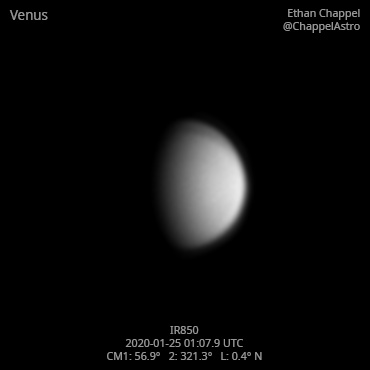# Venus 2020-01-25 01:07 UTC

CM1: 56.90°

CM2: 421.30°

CLat: +0.40°

Description

Pretty good night of imaging. Seeing was good, while transparency was excellent as usual for this time of year. Tonight was the first light of my new ZWO IR850 filter. I was hoping to see some details in the clouds with this filter, but it seems like that goal will have to wait.

Logs
```FireCapture v2.6  Settings
------------------------------------
Observer=Ethan Chappel
Camera=ZWO ASI174MM
Filter=IR
Profile=Venus
Diameter=14.71"
Magnitude=-4.07
FocalLength=7700mm (F/27)
Resolution=0.16"
Filename=2020-01-25-0109_8-EC-IR-Ven.ser
Date=2020_01_25
Start=01_08_20.471
Mid=01_09_50.473
End=01_11_20.476
Start(UT)=01_08_20.471
Mid(UT)=01_09_50.473
End(UT)=01_11_20.476
Duration=180.005s
Date_format=yyyy_MM_dd
Time_format=HH_mm_ss
LT=UT -6h
Frames captured=7792
File type=SER
Binning=no
Bit depth=8bit
Debayer=no
ROI=160x162
ROI(Offset)=0x0
FPS (avg.)=43
Shutter=5.000ms
Gain=345 (86%)
AutoGain=off
FPS=100 (off)
USBTraffic=80
Brightness=1 (off)
AutoExposure=off
Gamma=50 (off)
HighSpeed=off
SoftwareGain=10 (off)
AutoHisto=75 (off)
Histogramm(min)=0
Histogramm(max)=158
Histogramm=61%
Noise(avg.deviation)=1.36
AutoAlign=false
PreFilter=none
Limit=180 Seconds
Sensor temperature=28.3°C
FireCapture v2.6  Settings
------------------------------------
Observer=Ethan Chappel
Camera=ZWO ASI174MM
Filter=IR
Profile=Venus
Diameter=14.71"
Magnitude=-4.07
FocalLength=7700mm (F/27)
Resolution=0.16"
Filename=2020-01-25-0106_0-EC-IR-Ven.ser
Date=2020_01_25
Start=01_04_35.051
Mid=01_06_05.056
End=01_07_35.061
Start(UT)=01_04_35.051
Mid(UT)=01_06_05.056
End(UT)=01_07_35.061
Duration=180.010s
Date_format=yyyy_MM_dd
Time_format=HH_mm_ss
LT=UT -6h
Frames captured=7792
File type=SER
Binning=no
Bit depth=8bit
Debayer=no
ROI=160x162
ROI(Offset)=0x0
FPS (avg.)=43
Shutter=5.000ms
Gain=345 (86%)
AutoGain=off
FPS=100 (off)
USBTraffic=80
Brightness=1 (off)
AutoExposure=off
Gamma=50 (off)
HighSpeed=off
SoftwareGain=10 (off)
AutoHisto=75 (off)
Histogramm(min)=0
Histogramm(max)=154
Histogramm=60%
Noise(avg.deviation)=1.37
AutoAlign=false
PreFilter=none
Limit=180 Seconds
Sensor temperature=28.3°C
```